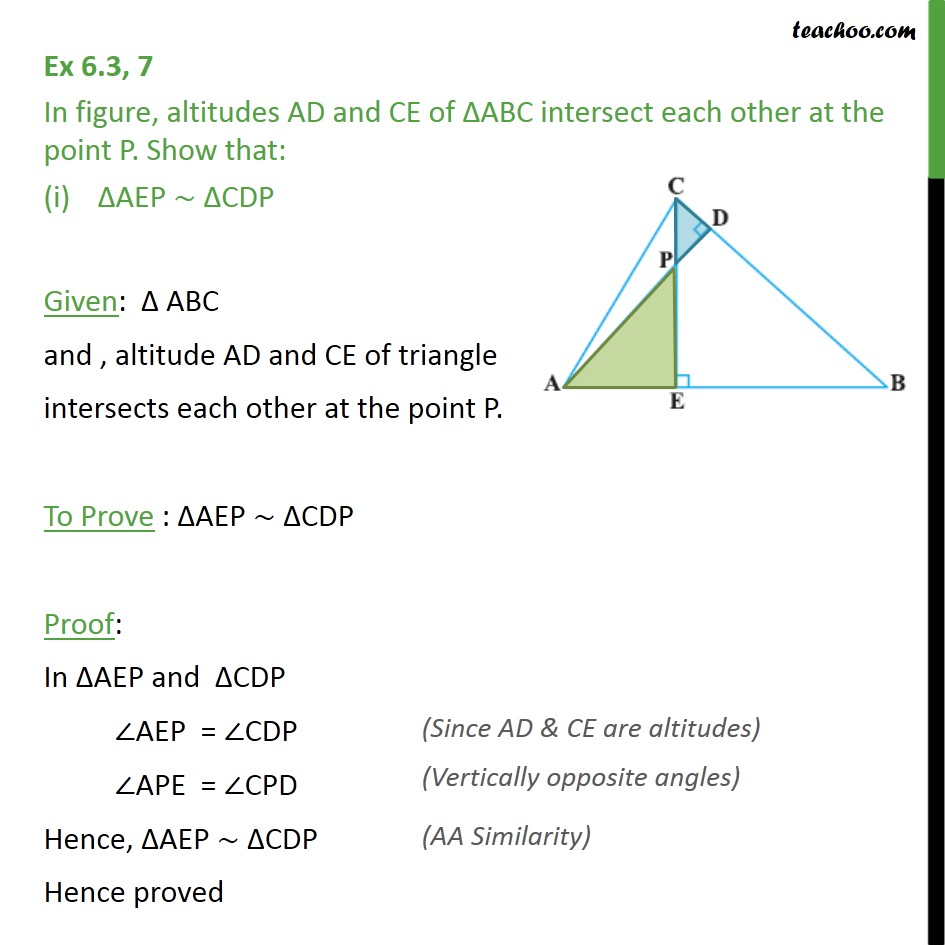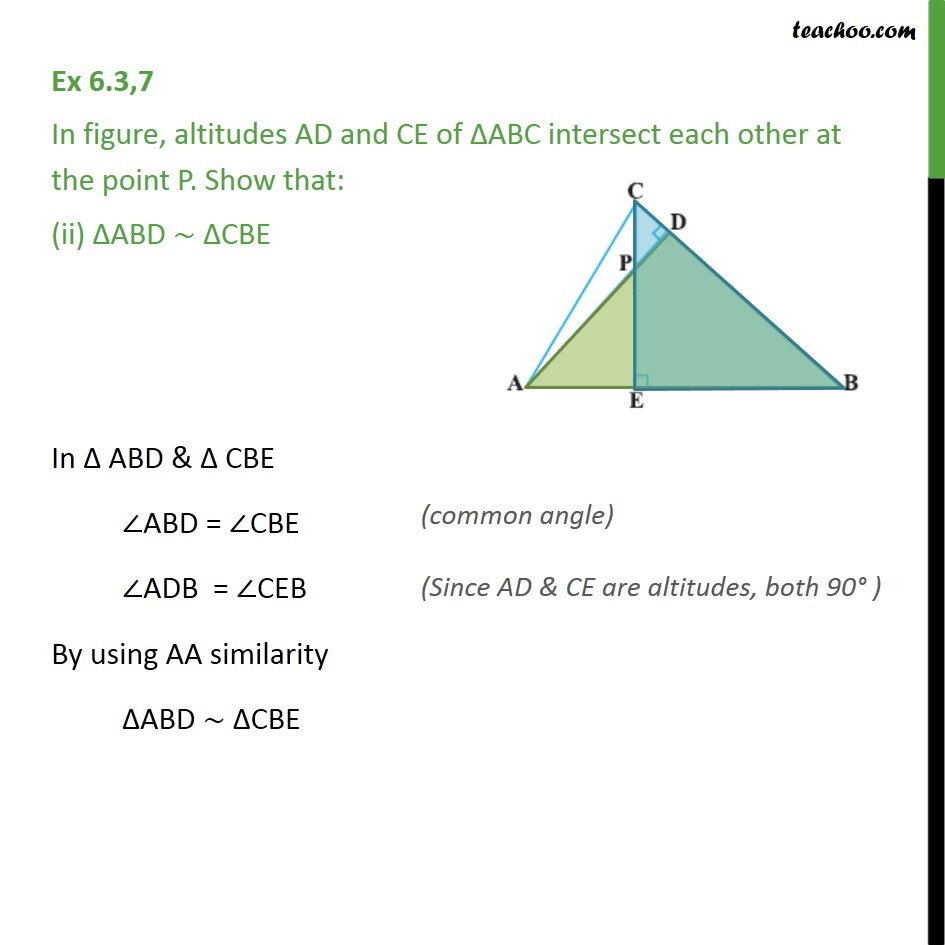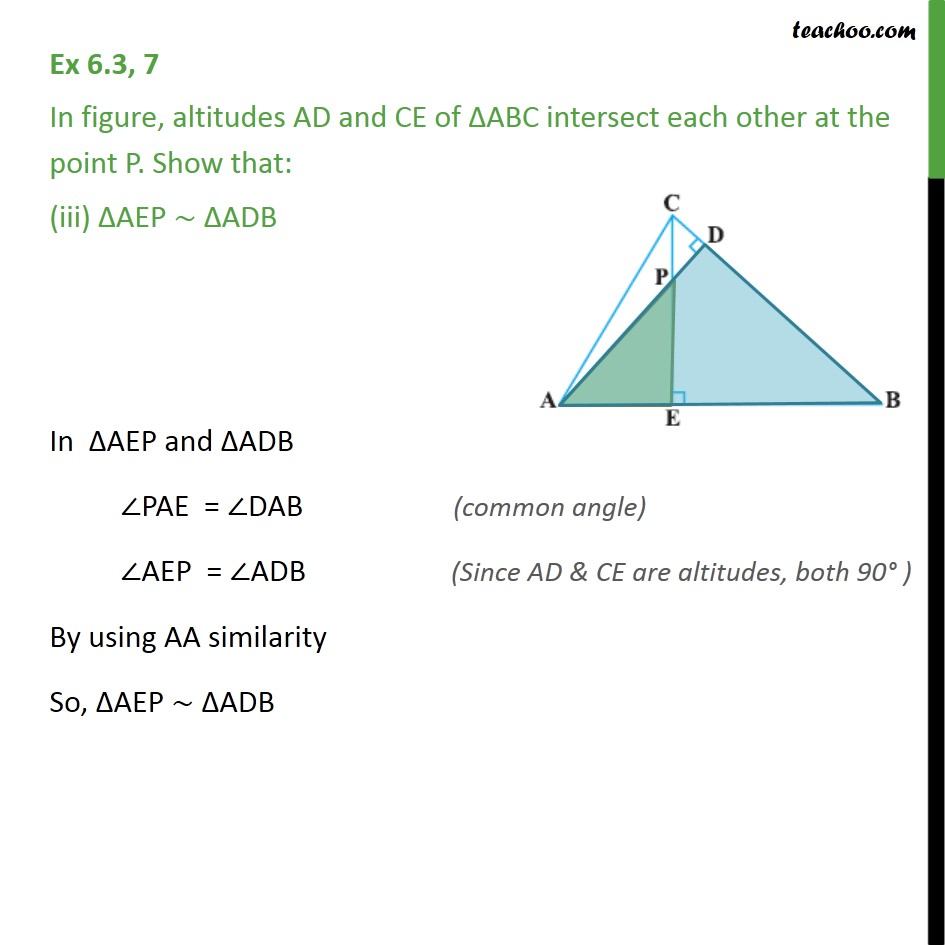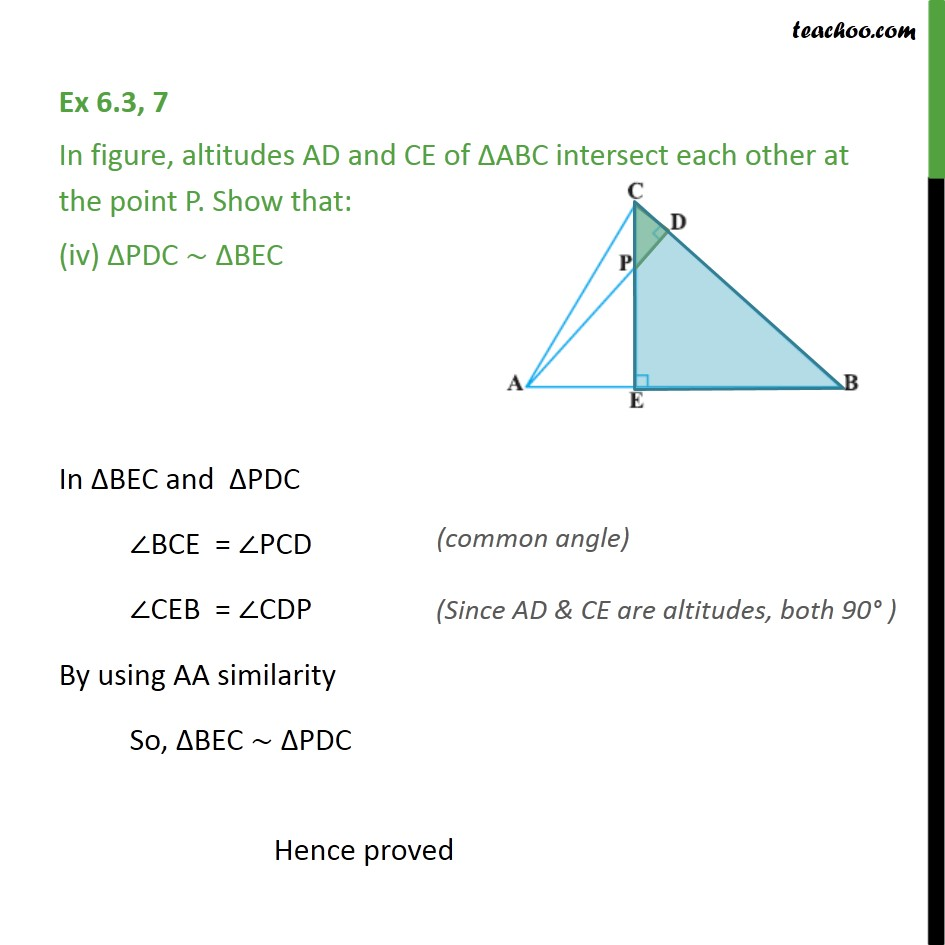Ex 6.3

Chapter 6 Class 10 Triangles
Serial order wiseLearn in your speed, with individual attention - Teachoo Maths 1-on-1 Class

### Transcript

Ex 6.3, 7 In figure, altitudes AD and CE of ΔABC intersect each other at the point P. Show that: ΔAEP ∼ ΔCDP Given: Δ ABC and , altitude AD and CE of triangle intersects each other at the point P. To Prove : ΔAEP ∼ ΔCDP Proof: In ΔAEP and ΔCDP ∠AEP = ∠CDP ∠APE = ∠CPD Hence, ΔAEP ∼ ΔCDP Hence proved Ex 6.3,7 In figure, altitudes AD and CE of ΔABC intersect each other at the point P. Show that: (ii) ΔABD ∼ ΔCBE In Δ ABD & Δ CBE ∠ABD = ∠CBE ∠ADB = ∠CEB By using AA similarity ΔABD ∼ ΔCBE Ex 6.3, 7 In figure, altitudes AD and CE of ΔABC intersect each other at the point P. Show that: (iii) ΔAEP ∼ ΔADB In ΔAEP and ΔADB ∠PAE = ∠DAB ∠AEP = ∠ADB By using AA similarity So, ΔAEP ∼ ΔADB Ex 6.3, 7 In figure, altitudes AD and CE of ΔABC intersect each other at the point P. Show that: (iv) ΔPDC ∼ ΔBEC In ΔBEC and ΔPDC ∠BCE = ∠PCD ∠CEB = ∠CDP By using AA similarity So, ΔBEC ∼ ΔPDC Hence proved